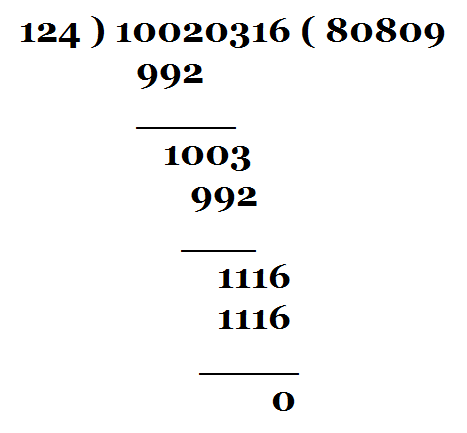# Solution

Where are the numbers? I can see only one 8 in the quotient and the final remainder is 0. All the rest are stars. How can I ever solve this!

Anyway, let me have a dash at it. First let me list out the things that we know:

1. The divisor is a three-digit number.

2. The dividend is an eight-digit number.

3. The quotient is a five-digit number with 8 in the hundreds place.

4. The final remainder is 0; this means the dividend is completely divisible by the divisor.

Now, observe that in the first step of the division, we get a three-digit number which when subtracted from the first four digits of the dividend leaves us with a two-digit number; the fifth digit from the dividend is then brought down but this proves to be inadequate and, so, the sixth digit of the dividend has also to be dropped down. Now, in order to drop down the sixth digit of the dividend, a zero needs to be added to the quotient. Thus, the quotient is of the form: *08**. We seem to be getting somewhere.

In the second step of the division, we again get a two-digit number as remainder and once again two digits (the seventh and eighth) of the dividend have to be dropped down. Two digits of the dividend can be brought down simultaneously only by adding a zero to the quotient. Therefore, the quotient is of the form: *080*. The quotient is looking somewhat respectable with only two stars giving it a scary look now.

Now, observe that in the second step of the division, the divisor when multiplied by 8 produced a three-digit number; in the final step, however, a four-digit number was required to produce 0 as the remainder. The ones digit in the quotient, therefore, has to be greater than 8. The only choice is 9. The quotient, therefore, is of the form: *0809.

The smallest three-digit number is 100. The smallest four-digit number is 1000. We have seen that the three-digit divisor when multiplied by 8 produces a three-digit number (less than 1000). Suppose we represent the divisor as "x", then 8x is less than 1000. This implies x is less than 125. Therefore, the divisor has to be a number from 100 to 124 (less than 125). This means the first digit of the divisor has to be 1. So, it is of the form: 1**.

In the final step of the division, the divisor when multiplied by 9 produces a four-digit number leaving the final remainder as 0. If the divisor were 111, then 111 x 9 = 999, a three-digit number. Therefore the divisor has to be greater than 111. So, we have narrowed our search for the divisor - it has to be number from 112 to 124.

We have seen that the divisor when multiplied by 8 produces a three-digit number and when multiplied by 9 produces a four-digit number. Therefore, the largest three-digit number it can yield is when it is multiplied by 8. In the first step of the division, we have a three-digit number that is subtracted from a four-digit number. This should be the largest three-digit number that the divisor can produce. Therefore, the first digit in the quotient has to be 8. Thus the quotient is 80809.

Alright, so what we have is: The quotient is 80809 and the divisor is one of the numbers from 112 to 124. The dividend is an eight-digit number. The remainder is 0. Therefore, divisor x quotient = dividend. Thus, 1** x 80809 = dividend (an eight-digit number). Let me give 123 a try as a divisor. 123 x 80809 = 9939507, a seven-digit number. Therefore, the divisor is greater than 123. The only choice is 124. The dividend is 124 x 80809 = 10020316 (an eight-digit number as required).

So, there, I have done it. The dividend is 10020316, the divisor is 124, and the quotient is 80809.

By the way, here are all the stars substituted by the actual digits:Well, I have spent hours trying to solve it and managed to get the answer after attacking the problem in my own clumsy manner. I am sure there must be a simpler and elegant way of solving it. I am sure you can work out an elegant and simpler solution.

• Union Public Service Commission - www.upsc.gov.in
• IIT-Kharagpur - www.iitkgp.ac.in
• Indian Statistical Institute - www.isical.ac.in
• Indian Institute of Technology Madras - www.iitm.ac.in
• Indian Institute of Management, Ahmedabad - www.iimahd.ernet.in
• Indian Institute of Mass Commission - www.iimc.nic.in
• IIT Bombay - www.iitb.ac.in